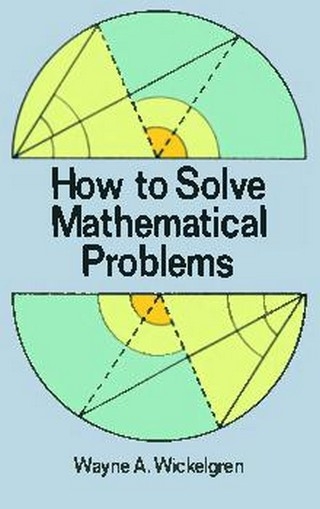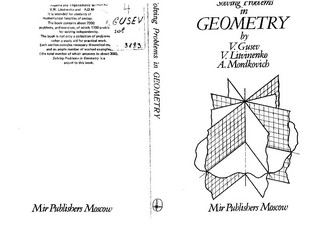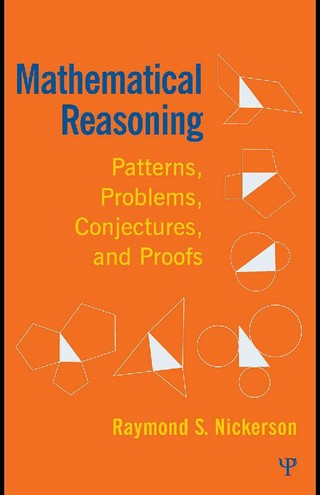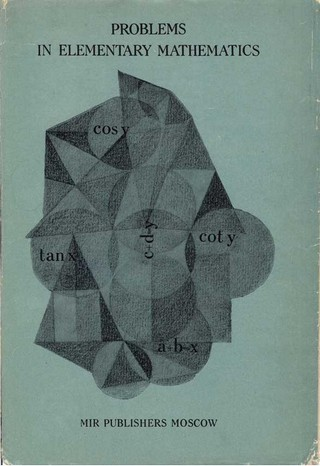### Book Toankho

Sách hay - Sách đẹp

Browsing:

# Tag: Problem

## The New Mathlete Problem Book (1961-1977 With Sample Solutions)The New Mathlete Problem Book (1961-1977 With Sample Solutions) Download ToanKho:http://lk.libvui.com/8UzhRoLb http://megaurl.io/9D3woO7

## How to Solve Mathematical ProblemsIf you’ve ever tried to solve mathematical problems without any idea how to go about it, this book is for you. It will improve your ability to solve all kinds of mathematical problems whether in mathematics, science, engineering, business, or Read more…

## Solving Mathematical Problems (A Personal Perspective)Authored by a leading name in mathematics, this engaging and clearly presented text leads the reader through the various tactics involved in solving mathematical problems at the Mathematical Olympiad level. Covering number theory, algebra, analysis, Euclidean geometry, and analytic geometry, Read more…

## Solving Problems in GeometrySolving Problems in Geometry, by V. Gusev, V. Litvinenko Download ToanKho:http://lk.libvui.com/qcJebf6p http://megaurl.io/TA30f

## Mathematical Reasoning (Patterns, Problems, Conjectures, and Proofs)The development of mathematical competence — both by humans as a species over millennia and by individuals over their lifetimes — is a fascinating aspect of human cognition. This book explores when and why the rudiments of mathematical capability first Read more…

## Problems-In-Elementary-Mathematics## The N. Y. City CONTEST PROBLEM BOOK (Prob & Sol from the N. Y. City Interscholastic Math League)Reproducible problems from the 1975-1984 New York City Interscholastic Mathematics League addressing Diophantine equations, polynomials, exponents, logarithms, complex numbers, motion problems, Pythagorean Theorem, combinatorics, sines and cosines, and more. Answers, solutions, appendixes, and bibliography. Download ToanKho:http://lk.libvui.com/9Btgbldj http://megaurl.in/FoIt

## A Problem Book in Real AnalysisEducation is an admirable thing, but it is well to remember from time to time that nothing worth knowing can be taught. Oscar Wilde, “The Critic as Artist,” 1890. Analysis is a profound subject; it is neither easy to understand Read more…